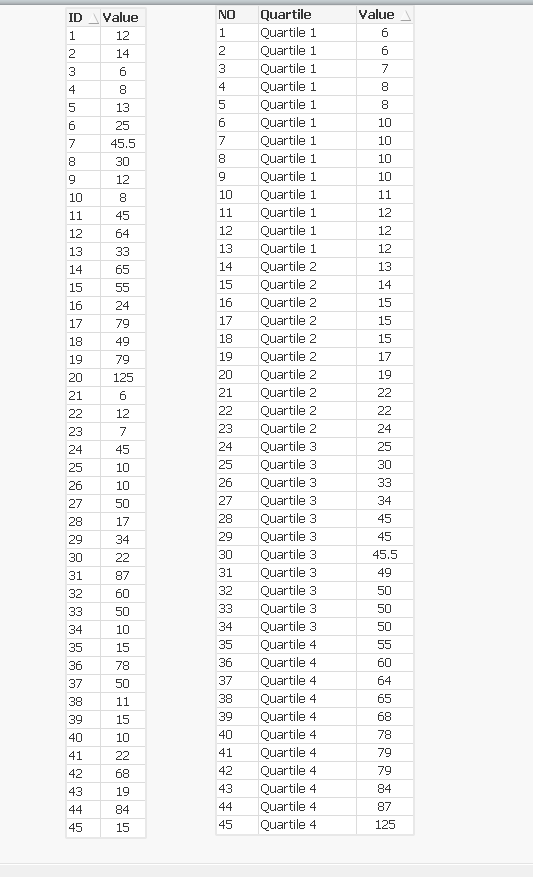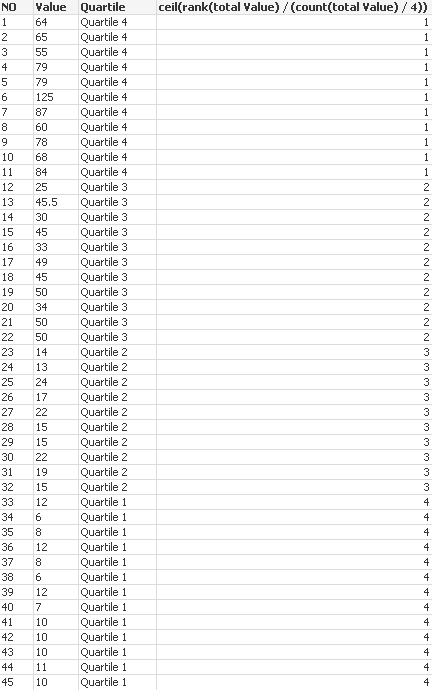# QlikView App Development

Discussion Board for collaboration related to QlikView App Development.

Announcements
Coming your way, the Qlik Data Revolution Virtual Summit. October 27-29. REGISTER
cancel
Showing results for
Did you mean:
HighlightedNot applicable

## How to distribute values into quartiles evently?

Hi Qlik folks,

I have a table of values which I aim to dividing it into Quartiles as evenly as possible. The expression I use in the chart is:

IF( Value <= Fractile (TOTAL Value, 0.25),

'Quartile 1',

IF( Value <= Fractile (TOTAL Value, 0.50),

'Quartile 2',

IF( Value <= Fractile (TOTAL Value, 0.75),

'Quartile 3',

'Quartile 4')))

This does the job, however the distribution of values is not even as I have equal values which my expression tends to put them all in one quartile.For the purpose of the analysis, I need the quartiles to have equal or at least near equal number of values. How can I achieve this?

Thanks,

Laleh

2 Replies
HighlightedMVP & Luminary

I think if you want to get a rather equal number of values within the buckets you will rather need a rank-function, maybe something like that:

ceil(rank(total Value) / (count(total Value) / 4))- Marcus

HighlightedPartner

The function I use to good effect is:

CEIL(RANK([Value]), 4) / COUNT(TOTAL [Value]) * 4)

The key being the RANK(Value, "Mode 4"): RANK Documentation